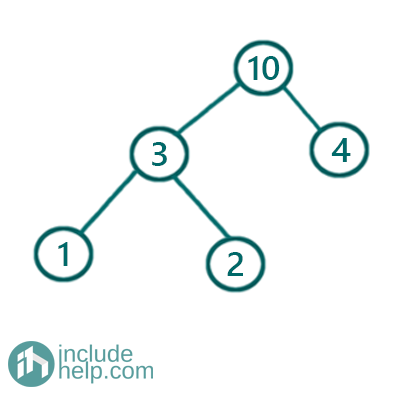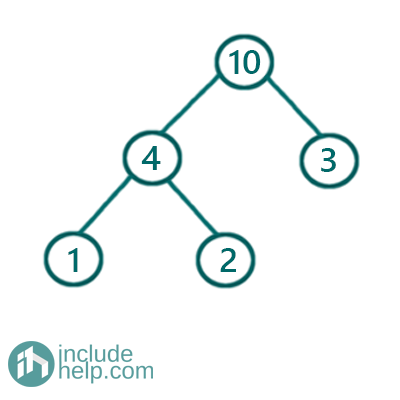# Check If a Given Tree is Sum Tree or Not

In this article, we are going to see how to check whether a tree is Sum tree or not?
Submitted by Radib Kar, on August 05, 2020

## Sum Tree

A sum tree is a tree whose root value is equal to the sum of left subtree & right subtree and whose subtrees are also sum tree.

We can validate which one is a sum tree and which one is not via examples:

1) A tree which is sumtreeThe above tree is a sum tree. We can check for each node and can finds that it maintains the constrains. For example if we consider the root, then root(10)=sum of left subtree(6)+ sum of right subtree(4) and also both subtrees are sum trees.

2) A tree which is not sumtreeThe above tree is not a sum tree as its left subtree is not a sumtree.

Solution

Naïve solution:

The first solution is the implementation of the naive idea which is to check for each node whether its subtrees are sumtree and the value of root is equal to sum of left subtree+sum of right subtree or not. Below is the implementation:

```#include <bits/stdc++.h>
using namespace std;

// tree node is defined
class TreeNode {
public:
int val;
TreeNode* left;
TreeNode* right;
TreeNode(int data)
{
val = data;
left = NULL;
right = NULL;
}
};

int sum(TreeNode* root)
{
if (!root)
return 0;
if (!root->left && !root->right)
return root->val;
return root->val + sum(root->left) + sum(root->right);
}

bool isSumTree(TreeNode* root)
{
if (!root)
return true;

if (!root->left && !root->right)
return true;

if (!(isSumTree(root->left) && isSumTree(root->right)))
return false;

int l = sum(root->left);
int r = sum(root->right);

return (root->val == (l + r));
}

int main()
{
//building the tree like example 1
TreeNode* root1 = new TreeNode(10);

root1->left = new TreeNode(3);
root1->right = new TreeNode(4);
root1->left->left = new TreeNode(1);
root1->left->right = new TreeNode(2);

if (isSumTree(root1))
cout << "Tree in example1 is a Sum Tree\n";
else
cout << "Tree in example1 is not a Sum Tree\n";

//building the tree-like example 2
TreeNode* root2 = new TreeNode(10);
root2->left = new TreeNode(4);
root2->right = new TreeNode(3);
root2->left->left = new TreeNode(1);
root2->left->right = new TreeNode(2);

if (isSumTree(root2))
cout << "Tree in example2 is a Sum Tree\n";
else
cout << "Tree in example2 is not a Sum Tree\n";

return 0;
}
```

Output:

```Tree in example1 is a Sum Tree
Tree in example2 is not a Sum Tree
```

In the above naïve approach, every time we computed the sum of the subtrees, which actually increases the time complexity to O(n^2) instead of O(n) and thus to reduce the time complexity to O(n) we have modified the above implementation and did like below:

Efficient Approach:

```#include <bits/stdc++.h>
using namespace std;

// tree node is defined
class TreeNode {
public:
int val;
TreeNode* left;
TreeNode* right;
TreeNode(int data)
{
val = data;
left = NULL;
right = NULL;
}
};

int sumTree(TreeNode* root)
{
if (!root)
return 0;

//if current node is leaf node return it's sum
if (!root->left && !root->right)
return root->val;

//left subtree sum
int l = sumTree(root->left);

//right subtree sum
int r = sumTree(root->right);

//if root val is equal to sum of left
//subtree + sum of right subtree then return the sum
if (root->val == l + r)
return root->val + l + r;
else //otherwise return INT_MIN
return INT_MIN;
}

bool isSumTree(TreeNode* root)
{
if (!root)
return true;
//if function returns INT_MIN then it's not a sumTree
if (sumTree(root) == INT_MIN)
return false;
return true;
}

int main()
{
//building the tree like example 1
TreeNode* root1 = new TreeNode(10);
root1->left = new TreeNode(3);
root1->right = new TreeNode(4);
root1->left->left = new TreeNode(1);
root1->left->right = new TreeNode(2);

if (isSumTree(root1))
cout << "Tree in example 1 is a Sum Tree\n";
else
cout << "Tree in example 1 is not a Sum Tree\n";

//building the tree-like example 2
TreeNode* root2 = new TreeNode(10);
root2->left = new TreeNode(4);
root2->right = new TreeNode(3);
root2->left->left = new TreeNode(1);
root2->left->right = new TreeNode(2);

if (isSumTree(root2))
cout << "Tree in example 2 is a Sum Tree\n";
else
cout << "Tree in example 2 is not a Sum Tree\n";

return 0;
}
```

Output:

```Tree in example 1 is a Sum Tree
Tree in example 2 is not a Sum Tree
```

What's New

Top Interview Coding Problems/Challenges!

Languages: » C » C++ » C++ STL » Java » Data Structure » C#.Net » Android » Kotlin » SQL
Web Technologies: » PHP » Python » JavaScript » CSS » Ajax » Node.js » Web programming/HTML
Solved programs: » C » C++ » DS » Java » C#
Aptitude que. & ans.: » C » C++ » Java » DBMS
Interview que. & ans.: » C » Embedded C » Java » SEO » HR
CS Subjects: » CS Basics » O.S. » Networks » DBMS » Embedded Systems » Cloud Computing
» Machine learning » CS Organizations » Linux » DOS
More: » Articles » Puzzles » News/Updates